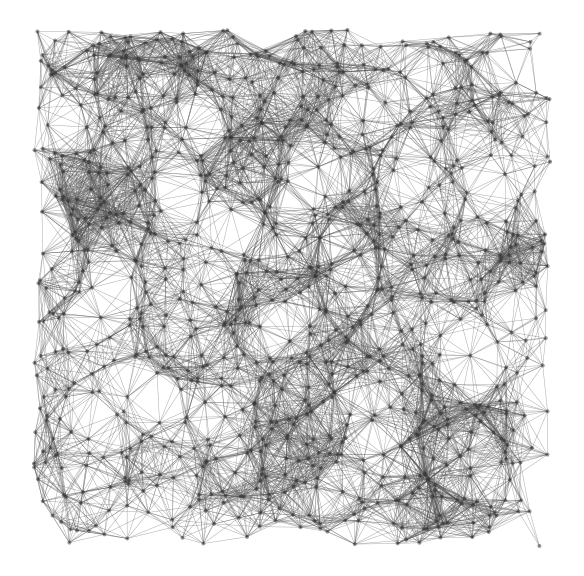# Random Euclidean graph generator

This generator creates random graphs of any size. Links of such graphs are created according to a threshold. If the Euclidean distance between two nodes is less than a given threshold, then a link is created between those 2 nodes.## Usage

Calling `begin()` put one unique node in the graph, then `nextEvents()` will add a new node each time it is called and connect this node to its neighbors according to the threshold planar Euclidean distance.

This generator has the ability to add randomly chosen numerical values on arbitrary attributes on edges or nodes of the graph, and to randomly choose a direction for edges.

A list of attributes can be given for nodes and edges. In this case each new node or edge added will have this attribute and the value will be a randomly chosen number. The range in which these numbers are chosen can be specified.

By default, edges are not oriented. It is possible to ask orientation, in which case the direction is chosen randomly.

By default, the graph is generated in the plane (2 dimensions) . Cartesian coordinates on nodes will be generated at random. So, each node will automatically be given two attributes: `x` and `y`. If a dimension is specified, then dimension attributes are generated, and the 2-norm distance Euclidean distance) is considered in that dimension between the nodes.

If the dimension is 2, then attributes `x` and `y` are defined for each node. If dimension is 3, then attributes `x`, `y` and `z` are used. For other values of dimension, dimension attributes are defined (`xi` with `i` in dimension) .

## Complexity

For the construction of a n nodes graph, the complexity is about O(n^2).

## Example

Here is a simple example of use: